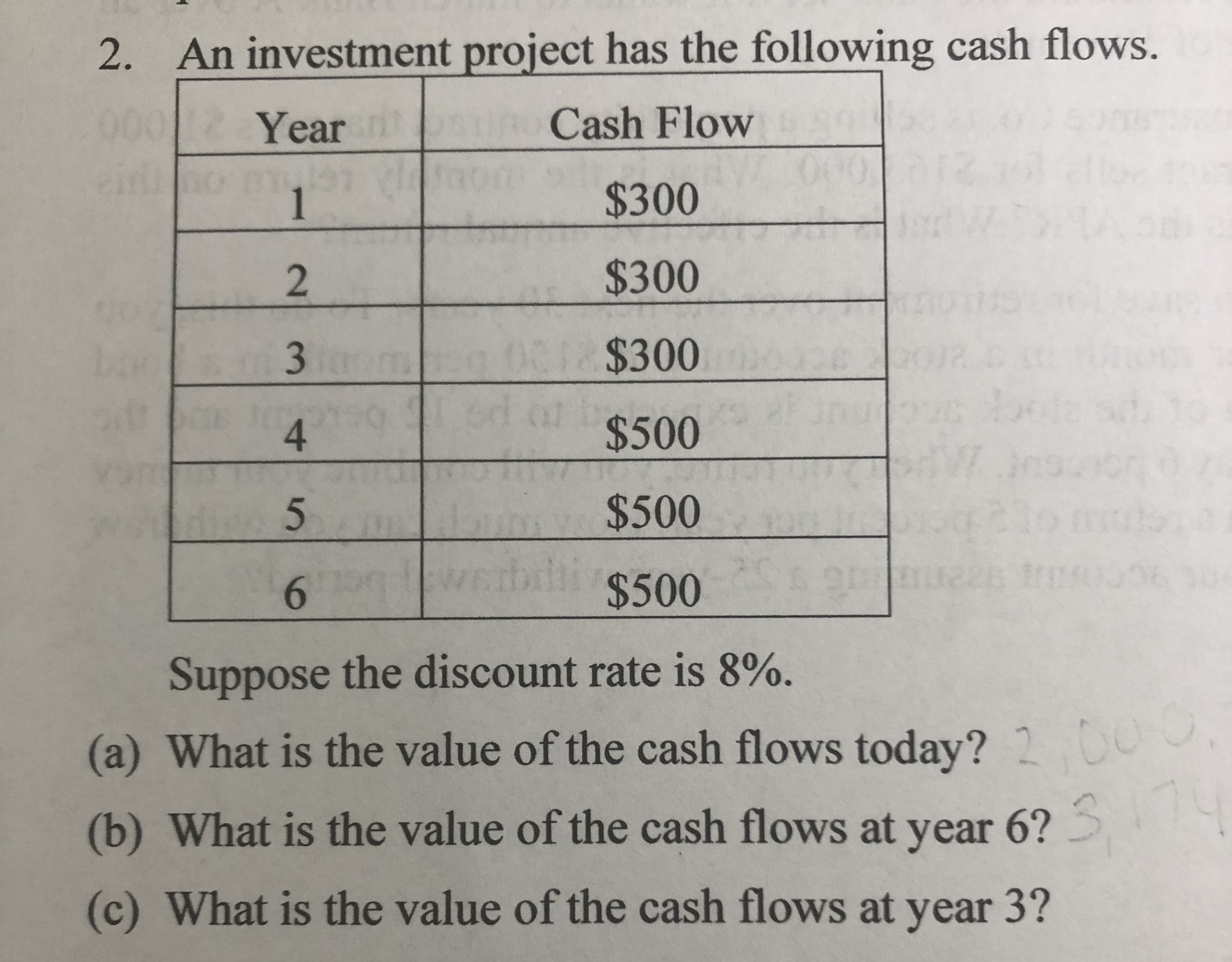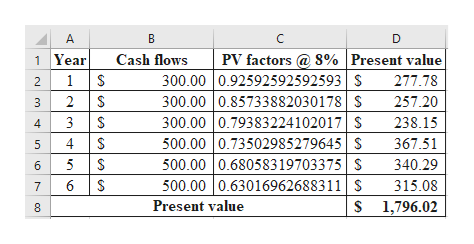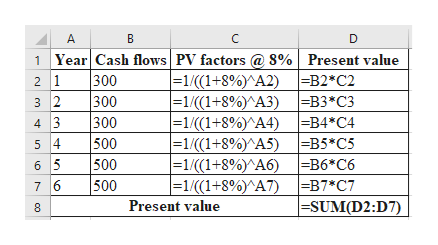# An investment project has the following cash flows.2.001 :Cash FlowYear060\$3001\$300201 \$30034F\$5004\$50021bdl \$5006Suppose the discount rate is 8%.(a) What is the value of the cash flows today? 20014(b) What is the value of the cash flows at year 6?(c) What is the value of the cash flows at year 3?3E

Question
34 viewshelp_outlineImage TranscriptioncloseAn investment project has the following cash flows. 2. 001 : Cash Flow Year 060 \$300 1 \$300 2 01 \$300 3 4F \$500 4 \$500 21 bdl \$500 6 Suppose the discount rate is 8%. (a) What is the value of the cash flows today? 2 00 14 (b) What is the value of the cash flows at year 6? (c) What is the value of the cash flows at year 3? 3E fullscreen
check_circle

Step 1

1.

Calculate the present value as follows:help_outlineImage TranscriptioncloseА В C D PV factors S% |Present value 300.00 0.92592592592593 S 300.00 0.85733882030178S 300.00 0.79383224102017 500.00 0.73502985279645S 500.00 0.68058319703375S 500.00 0.63016962688311S 1 Year Cash flows 2 1 277.78 2 257.20 3 3 238.15 4 \$ 367.51 5 340.29 6 315.08 7 S 1,796.02 Present value n LO fullscreen
Step 2

Workings:help_outlineImage TranscriptioncloseA 1 Year Cash flows PV factors @ 8% ] Present value 2 1 -B2*C2 |-1/ ( (1+8%)^A2) |300 300 =1/((1+8%)^A3) B3*C3 3 2 300 |=1/((1+8%)^A4) =B4*C4 4 3 500 = 1 / (( 1+8%)^A5) 5 4 B5*C5 500 =1/((1+8%)^A6) B6*C6 6 5 500 |- 1/ ((1+8%)^A7) 7 6 -B7*C7 |-SUM(D2:D7) Present value fullscreen
Step 3

2.

Calculate the value at ye...

### Want to see the full answer?

See Solution

#### Want to see this answer and more?

Solutions are written by subject experts who are available 24/7. Questions are typically answered within 1 hour.*

See Solution
*Response times may vary by subject and question.
Tagged in